|

# 这个94年帅哥颜值爆表,在湖边爆改3层小白楼,却只愿做一个48小时贴身管家

你想要的家是什么样的

有雨后森林嫩芽带着的泥土味

有吹过大海拂过山谷的清风气息

还有贴心的他

于盘旋湖心的小白船上微笑示意

“走吧，跟我回家”李唯，大家都叫他Lee

低调网红

不但是只94年的头牌小鲜肉

而且貌美肤白大长腿

曾在宁波一家精品酒店里做管家作为店里的颜值担当兼前台小哥

他学会调酒、煮咖啡、泡茶、烧海鲜

陪南来北往的人聊天

为他们挑选书、音乐和电影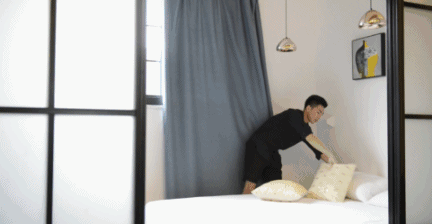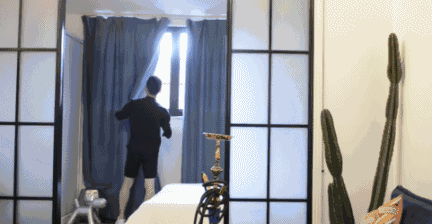漂亮的双手用来冲咖啡滋味比谁都鲜美

全身散发着很想红的低调

他帮助了许多南来北往的陌生人

让他们在陌生的城市里找到了家的安心和舒适！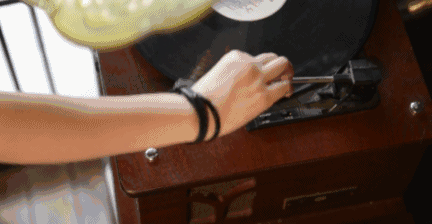然而当初做民宿管家

他只是想换个环境工作

没想到从此就爱上了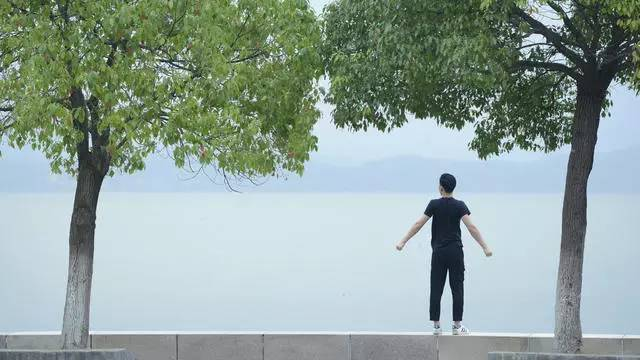世界上落脚的酒店那么多

住得像家的没几个

渐渐地

Lee忍不住想要做一栋自己的民宿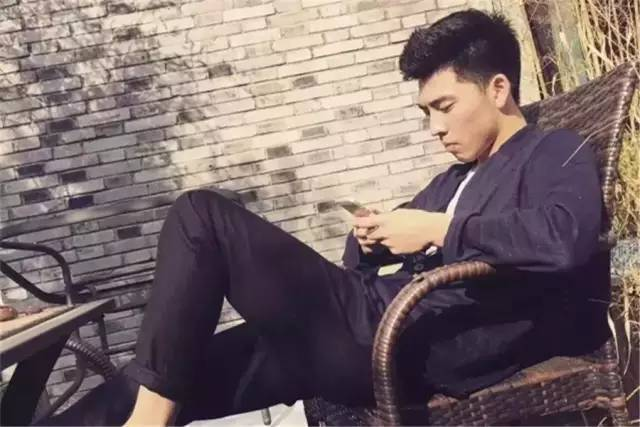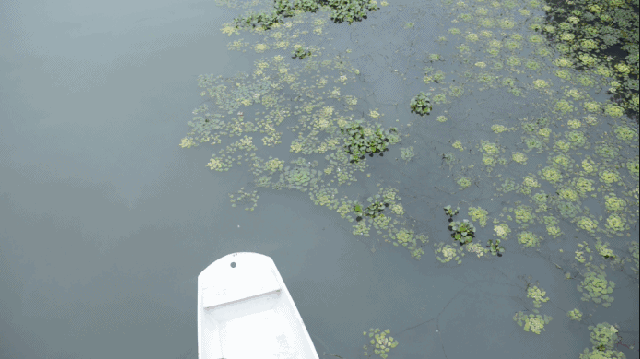机缘巧合下

Lee竟真的遇到了这样一座3层小楼

小楼位于宁波东钱湖畔

离东钱湖乐园不足10分钟的车程

却是远离尘嚣作为一个水瓶男

Lee坚持自己亲手装饰小白屋

油漆、粉刷、搬家具、运花盆、做摆件

从山上捡来树枝钉在墙上做装饰

再用大大小小的绿色植物摆满房间

搭配大量的绿植盆栽原木家具，清新简洁粉刷小白屋

房子有了，那还等什么

年轻人说干就干

油漆、粉刷、搬家具、运花盆、做摆件

全都自己动手从山上捡来树枝钉在墙上做装饰

再用大大小小的绿色植物摆满房间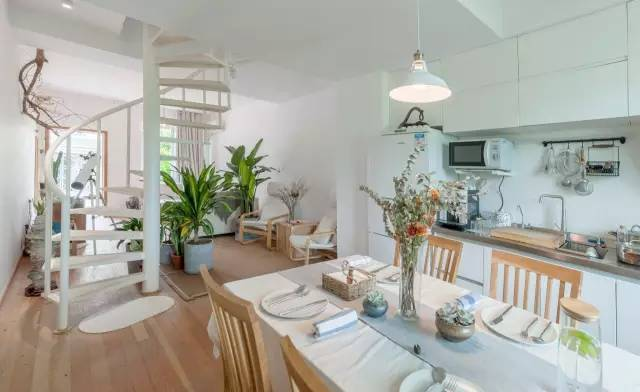经过两个月的时间

原本空荡荡的房子，有了雏形

可算是有个家的模样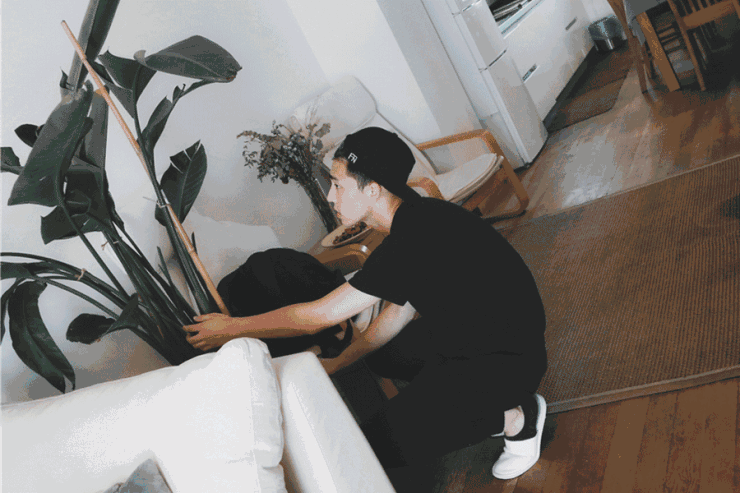2个月时间，空荡荡的房子

就盛开成一朵生机勃勃的“野合”了

小白屋是大名

它还有个小名叫“野合”

取“野百合也有春天”之意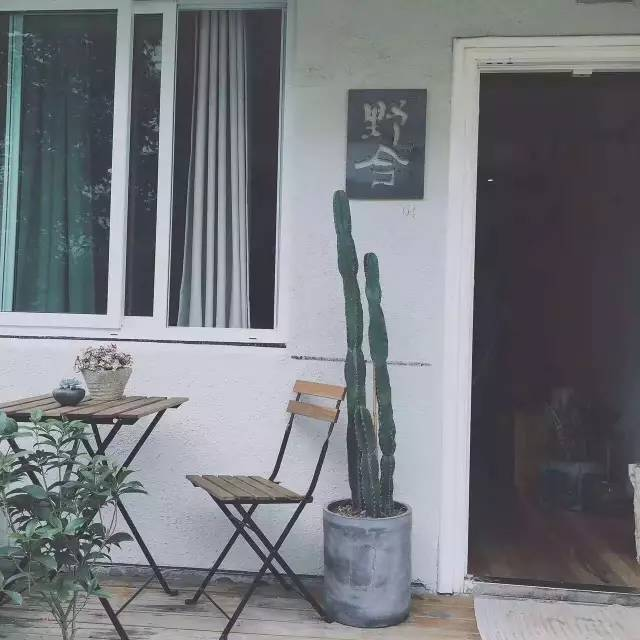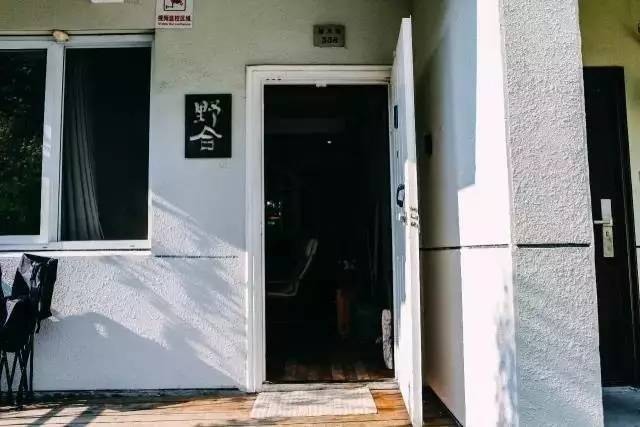小白屋的室内设计师孙阳光

对，她就叫阳光

深邃的眸子、五官立体

Lee喜欢叫她“乌克兰美女”小白屋的风格定位与软装

都是阳光带来的这个仅仅只有110平方米的三层空间

整个空间以白色为主色调

所有陈设都像是没有精心考虑的随性所为

其实每一处都是他们改过好多次的

符合小白屋感觉的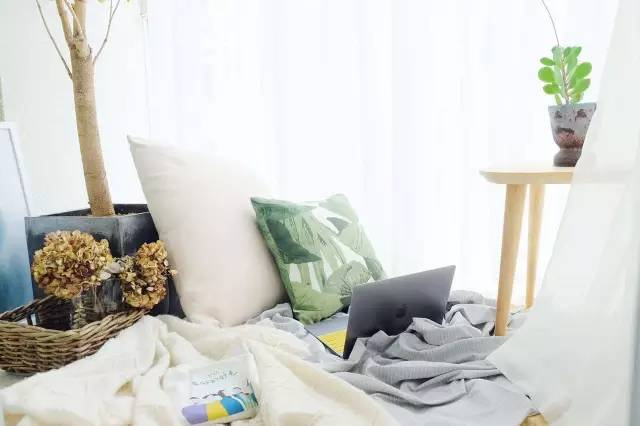整个装修过程都没有图纸

定期去现场调整

他们希望它更像家，更自然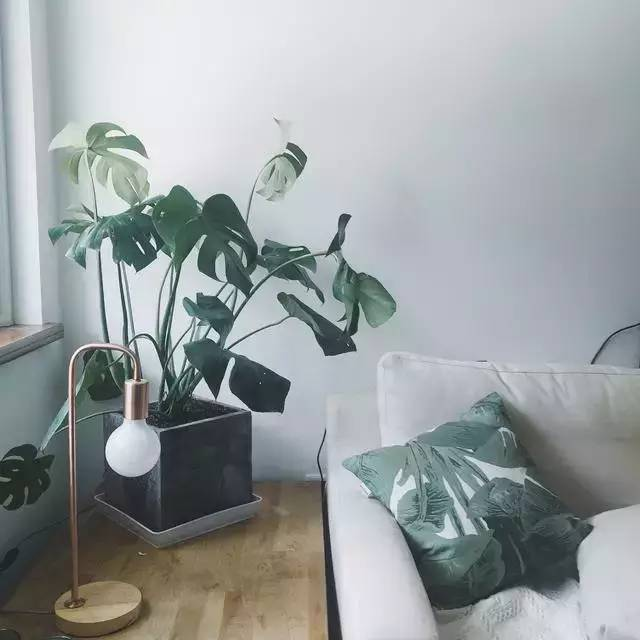搭配大量的绿植盆栽

原木家具、地板，清新简洁

门口有个迷你的院落

种着各色植物

另用白沙铺地作为景观的一部分

在这与家人喝茶、闲聊、吹风再也适合不过

晌午的阳光被枝叶划分得细细碎碎的

光影落得满地都是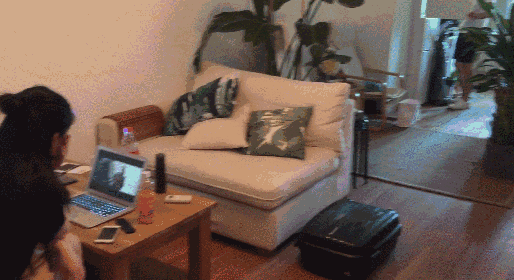进门，一个精致的客厅展现在眼前

在这个不太大的空间里

在设计上花了更多心思

也带来惊喜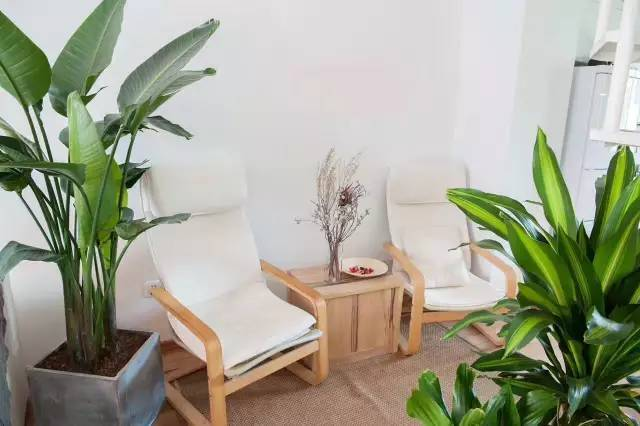原木地板、家具

搭配着绿植盆栽和白色墙面

简洁清新像一位画了淡妆的少女

屋主一定是在和房子谈一场恋爱整个空间以白色为主色调

趣味的小装饰做点缀

低调中却不失眼前一亮的惊喜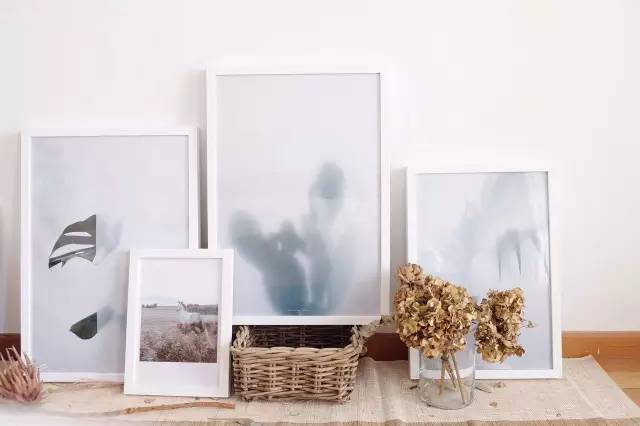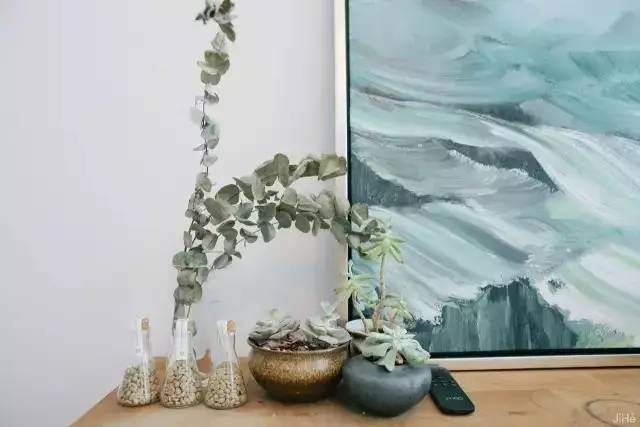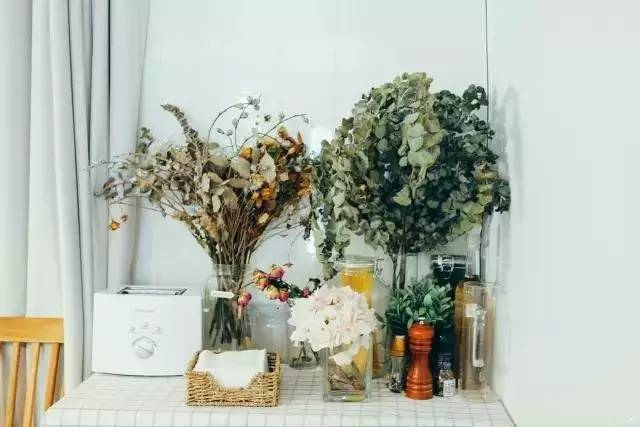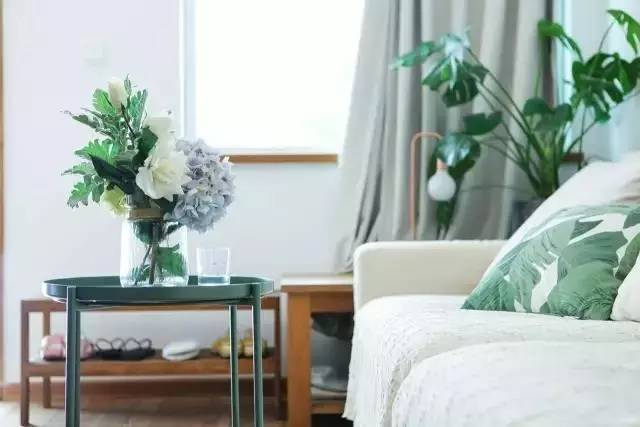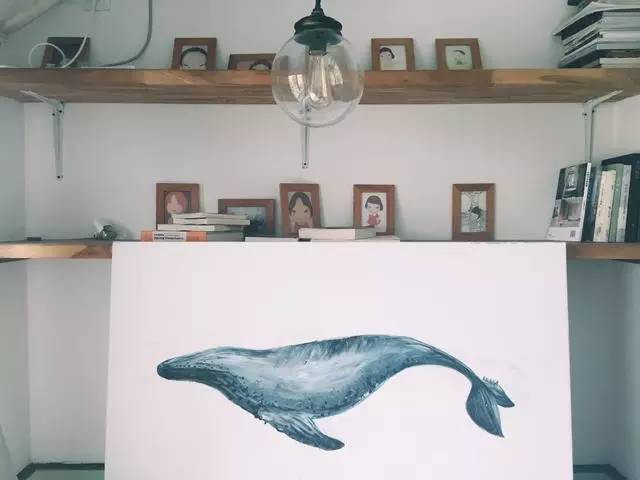桌子和地板上的干花、阁楼的书架

与奈良美智（Yoshitomo Nara）的小女孩

都是阳光带来的大量的艺术装饰品

让这个明亮通透的空间看起来更为饱满

壁画装饰了单调的空间

艺术装饰品的堆积

犹如空气组成

使整个空间似乎穿透他又无法摆脱它

看似极简的风格却在细节上

强调了功能与美感的平衡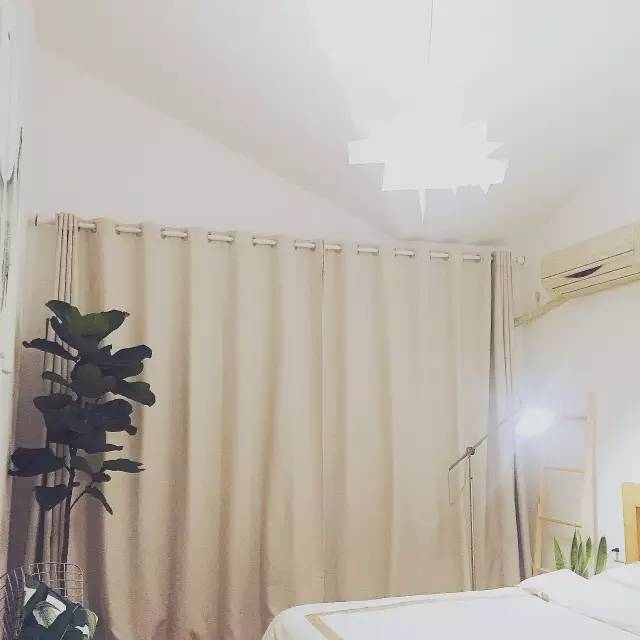卧室也以白色基调为主

在视觉上开拓了空间

利用干净利落的装饰物品使白色更有层次感

明亮通透的光线照射进来

舒适宜人阳光为了阳光能充满小白屋

把它改得像温室花房

大窗看出去就是湖景二楼卧室同样以白色基调为主

在视觉上开拓了空间

利用艺术品装饰物品使白色更有层次感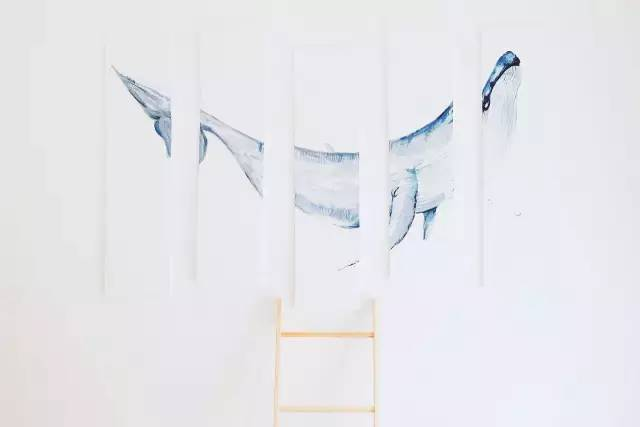原木的桌椅搭配着鲜花绿植

营造了一个温馨的就餐环境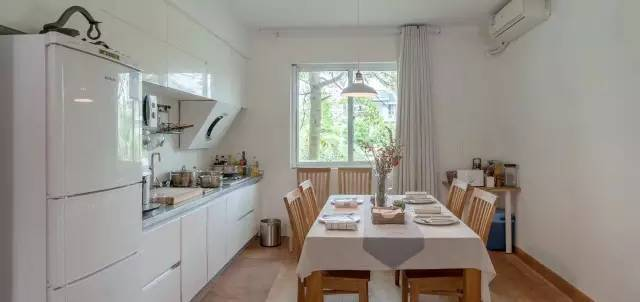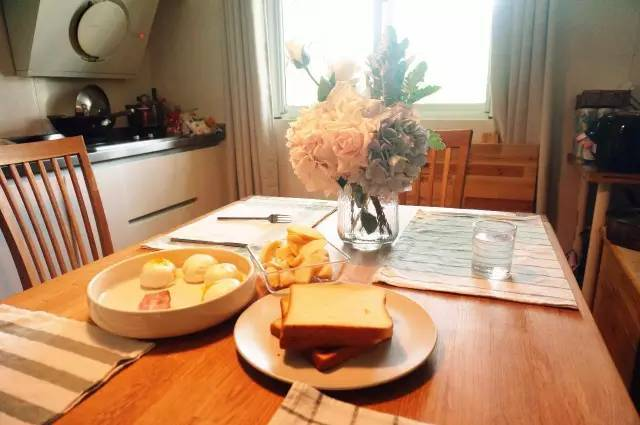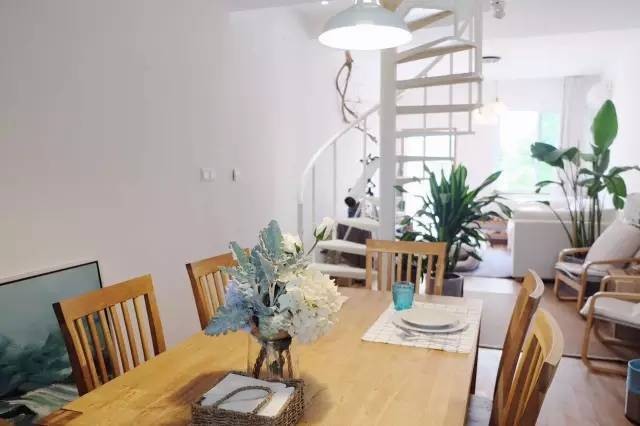柔和灯光下充满食欲的餐厅

鲜花搭配诱人的食物

穿插着日常美好

这便是这个居所的美妙之处素色沙发配矮茶几

纯白墙壁配复古木地板

以及分外抢眼的大长腿

不禁令人感慨住在这里是多么幸福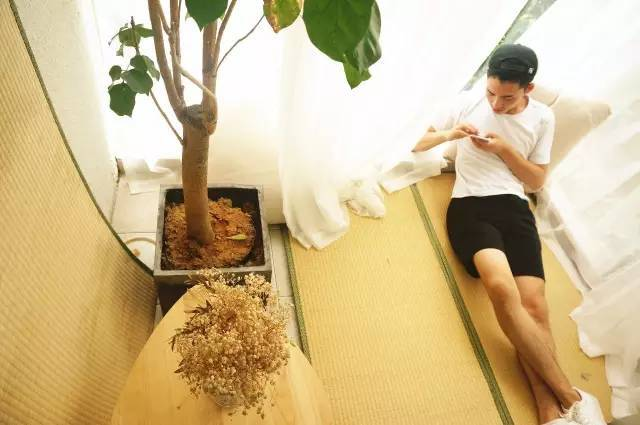不想被打扰的周末

可以在小白屋睡到自然醒

午后在阳台的榻榻米上伸个懒腰

像植物一样进行下光合作用可以从后院划小船去游湖

当然船也是白色的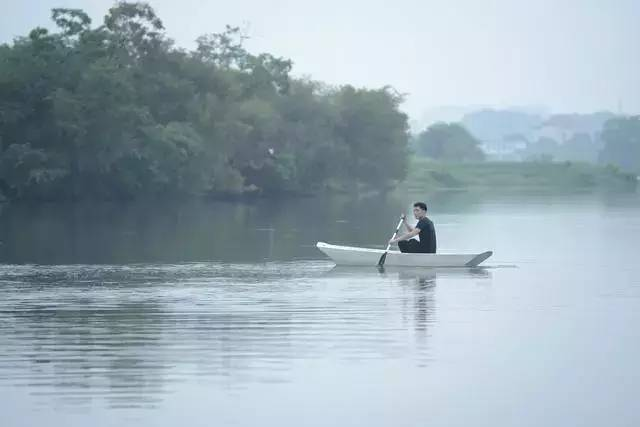小白屋还有个私密空间，地下密室

如果你能找到入口

就能进入到他的私家影院里

躺着趴着什么姿势都不介意

选一部老片子细细品味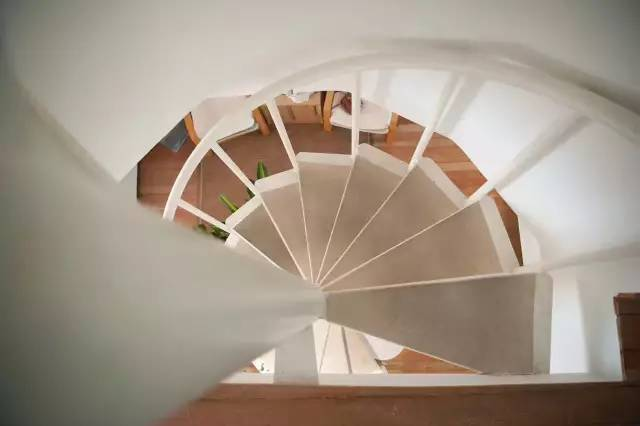或者

返身从白得发光的旋转木梯

爬向星空下的床

看书、听歌、小憩一会

就这样慵懒睡去也挺好，或者

返身从白得发光的旋转木梯上楼

回到星空下的床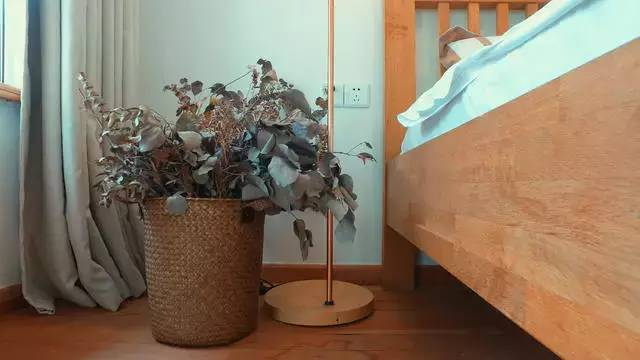当你看到海淘爆款Marvis牙膏

镜面梳妆台上满满一盒资生堂化妆棉

你就知道他的朋友们为什么叫他“李大仁”了

作为最懂女人心的直男

他想提醒各位姑娘，再累

也要记得仔细卸妆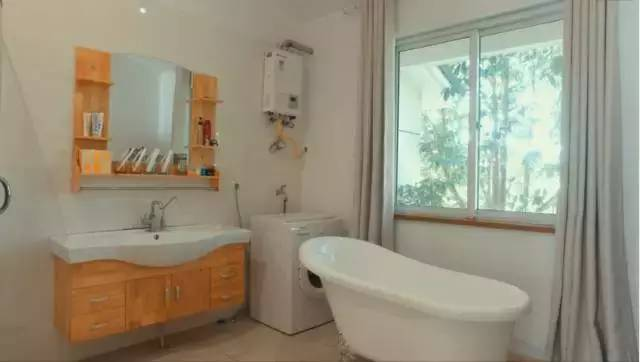再懒也要吃个Brunch

然后下午才有动力

荡起双桨从后院起航嘛

不管一个人、两个人

友谊的小船不会说翻就翻

这可是感情的巨轮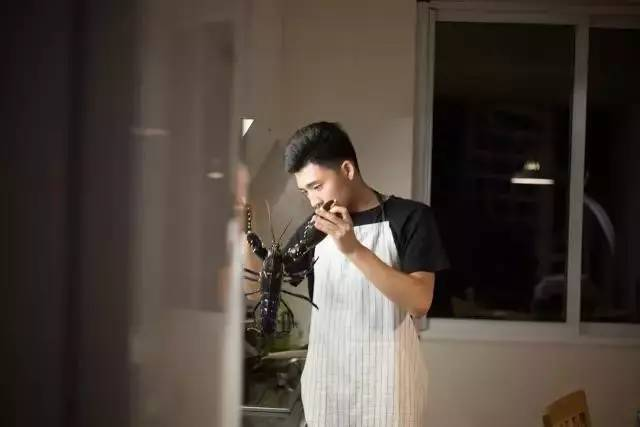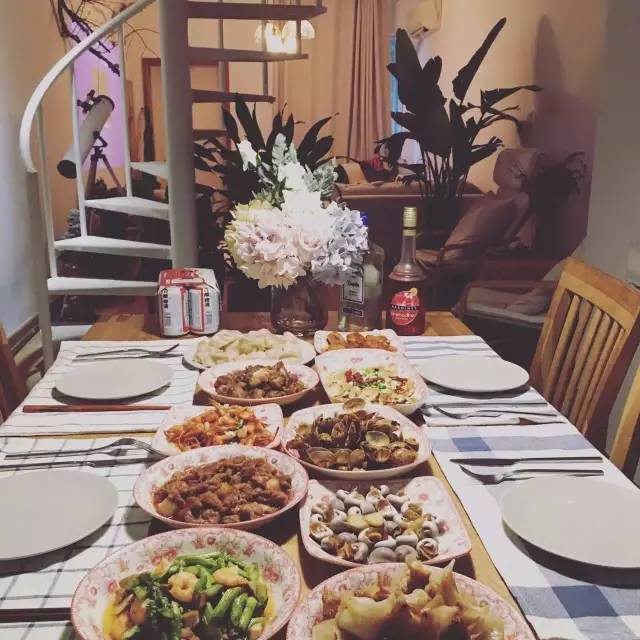傍晚到家

地道的宁波家常菜

还有舟山大青山活送海鲜已经满桌候着了

开放式厨房里的烹调用具和调料一应俱全

你也可以自己露一手晚上

走出窗外跨坐在水泥底的瓦片上

喝点小酒吹吹风

坐等几小时后的阳光叫醒自己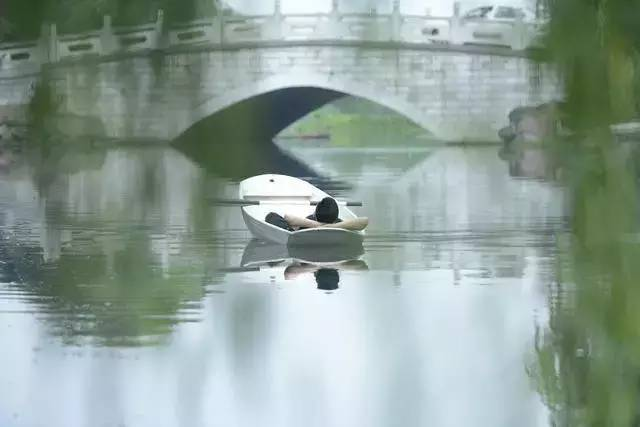世界充满了无数的可能

整理心情，重新出发

可能遇见另一种人生

惊喜，就在下一个转角

`声明：本文由入驻焦点开放平台的作者撰写，除焦点官方账号外，观点仅代表作者本人，不代表焦点立场错误信息举报电话： 400-099-0099，邮箱：jubao@vip.sohu.com，或点此进行意见反馈，或点此进行举报投诉。`A B C D E F G H J K L M N P Q R S T W X Y Z
A - B - C - D - E
• A
• 鞍山
• 安庆
• 安阳
• 安顺
• 安康
• 澳门
• B
• 北京
• 保定
• 包头
• 巴彦淖尔
• 本溪
• 蚌埠
• 亳州
• 滨州
• 北海
• 百色
• 巴中
• 毕节
• 保山
• 宝鸡
• 白银
• 巴州
• C
• 承德
• 沧州
• 长治
• 赤峰
• 朝阳
• 长春
• 常州
• 滁州
• 池州
• 长沙
• 常德
• 郴州
• 潮州
• 崇左
• 重庆
• 成都
• 楚雄
• 昌都
• 慈溪
• 常熟
• D
• 大同
• 大连
• 丹东
• 大庆
• 东营
• 德州
• 东莞
• 德阳
• 达州
• 大理
• 德宏
• 定西
• 儋州
• 东平
• E
• 鄂尔多斯
• 鄂州
• 恩施
F - G - H - I - J
• F
• 抚顺
• 阜新
• 阜阳
• 福州
• 抚州
• 佛山
• 防城港
• G
• 赣州
• 广州
• 桂林
• 贵港
• 广元
• 广安
• 贵阳
• 固原
• H
• 邯郸
• 衡水
• 呼和浩特
• 呼伦贝尔
• 葫芦岛
• 哈尔滨
• 黑河
• 淮安
• 杭州
• 湖州
• 合肥
• 淮南
• 淮北
• 黄山
• 菏泽
• 鹤壁
• 黄石
• 黄冈
• 衡阳
• 怀化
• 惠州
• 河源
• 贺州
• 河池
• 海口
• 红河
• 汉中
• 海东
• I
• J
• 晋中
• 锦州
• 吉林
• 鸡西
• 佳木斯
• 嘉兴
• 金华
• 景德镇
• 九江
• 吉安
• 济南
• 济宁
• 焦作
• 荆门
• 荆州
• 江门
• 揭阳
• 金昌
• 酒泉
• 嘉峪关
K - L - M - N - P
• K
• 开封
• 昆明
• 昆山
• L
• 廊坊
• 临汾
• 辽阳
• 连云港
• 丽水
• 六安
• 龙岩
• 莱芜
• 临沂
• 聊城
• 洛阳
• 漯河
• 娄底
• 柳州
• 来宾
• 泸州
• 乐山
• 六盘水
• 丽江
• 临沧
• 拉萨
• 林芝
• 兰州
• 陇南
• M
• 牡丹江
• 马鞍山
• 茂名
• 梅州
• 绵阳
• 眉山
• N
• 南京
• 南通
• 宁波
• 南平
• 宁德
• 南昌
• 南阳
• 南宁
• 内江
• 南充
• P
• 盘锦
• 莆田
• 平顶山
• 濮阳
• 攀枝花
• 普洱
• 平凉
Q - R - S - T - W
• Q
• 秦皇岛
• 齐齐哈尔
• 衢州
• 泉州
• 青岛
• 清远
• 钦州
• 黔南
• 曲靖
• 庆阳
• R
• 日照
• 日喀则
• S
• 石家庄
• 沈阳
• 双鸭山
• 绥化
• 上海
• 苏州
• 宿迁
• 绍兴
• 宿州
• 三明
• 上饶
• 三门峡
• 商丘
• 十堰
• 随州
• 邵阳
• 韶关
• 深圳
• 汕头
• 汕尾
• 三亚
• 三沙
• 遂宁
• 山南
• 商洛
• 石嘴山
• T
• 天津
• 唐山
• 太原
• 通辽
• 铁岭
• 泰州
• 台州
• 铜陵
• 泰安
• 铜仁
• 铜川
• 天水
• 天门
• W
• 乌海
• 乌兰察布
• 无锡
• 温州
• 芜湖
• 潍坊
• 威海
• 武汉
• 梧州
• 渭南
• 武威
• 吴忠
• 乌鲁木齐
X - Y - Z
• X
• 邢台
• 徐州
• 宣城
• 厦门
• 新乡
• 许昌
• 信阳
• 襄阳
• 孝感
• 咸宁
• 湘潭
• 湘西
• 西双版纳
• 西安
• 咸阳
• 西宁
• 仙桃
• 西昌
• Y
• 运城
• 营口
• 盐城
• 扬州
• 鹰潭
• 宜春
• 烟台
• 宜昌
• 岳阳
• 益阳
• 永州
• 阳江
• 云浮
• 玉林
• 宜宾
• 雅安
• 玉溪
• 延安
• 榆林
• 银川
• Z
• 张家口
• 镇江
• 舟山
• 漳州
• 淄博
• 枣庄
• 郑州
• 周口
• 驻马店
• 株洲
• 张家界
• 珠海
• 湛江
• 肇庆
• 中山
• 自贡
• 资阳
• 遵义
• 昭通
• 张掖
• 中卫

1室1厅1厨1卫1阳台

1
2
3
4
5

0
1
2

1

1

0
1
2
3报名成功，资料已提交审核A B C D E F G H J K L M N P Q R S T W X Y Z
A - B - C - D - E
• A
• 鞍山
• 安庆
• 安阳
• 安顺
• 安康
• 澳门
• B
• 北京
• 保定
• 包头
• 巴彦淖尔
• 本溪
• 蚌埠
• 亳州
• 滨州
• 北海
• 百色
• 巴中
• 毕节
• 保山
• 宝鸡
• 白银
• 巴州
• C
• 承德
• 沧州
• 长治
• 赤峰
• 朝阳
• 长春
• 常州
• 滁州
• 池州
• 长沙
• 常德
• 郴州
• 潮州
• 崇左
• 重庆
• 成都
• 楚雄
• 昌都
• 慈溪
• 常熟
• D
• 大同
• 大连
• 丹东
• 大庆
• 东营
• 德州
• 东莞
• 德阳
• 达州
• 大理
• 德宏
• 定西
• 儋州
• 东平
• E
• 鄂尔多斯
• 鄂州
• 恩施
F - G - H - I - J
• F
• 抚顺
• 阜新
• 阜阳
• 福州
• 抚州
• 佛山
• 防城港
• G
• 赣州
• 广州
• 桂林
• 贵港
• 广元
• 广安
• 贵阳
• 固原
• H
• 邯郸
• 衡水
• 呼和浩特
• 呼伦贝尔
• 葫芦岛
• 哈尔滨
• 黑河
• 淮安
• 杭州
• 湖州
• 合肥
• 淮南
• 淮北
• 黄山
• 菏泽
• 鹤壁
• 黄石
• 黄冈
• 衡阳
• 怀化
• 惠州
• 河源
• 贺州
• 河池
• 海口
• 红河
• 汉中
• 海东
• I
• J
• 晋中
• 锦州
• 吉林
• 鸡西
• 佳木斯
• 嘉兴
• 金华
• 景德镇
• 九江
• 吉安
• 济南
• 济宁
• 焦作
• 荆门
• 荆州
• 江门
• 揭阳
• 金昌
• 酒泉
• 嘉峪关
K - L - M - N - P
• K
• 开封
• 昆明
• 昆山
• L
• 廊坊
• 临汾
• 辽阳
• 连云港
• 丽水
• 六安
• 龙岩
• 莱芜
• 临沂
• 聊城
• 洛阳
• 漯河
• 娄底
• 柳州
• 来宾
• 泸州
• 乐山
• 六盘水
• 丽江
• 临沧
• 拉萨
• 林芝
• 兰州
• 陇南
• M
• 牡丹江
• 马鞍山
• 茂名
• 梅州
• 绵阳
• 眉山
• N
• 南京
• 南通
• 宁波
• 南平
• 宁德
• 南昌
• 南阳
• 南宁
• 内江
• 南充
• P
• 盘锦
• 莆田
• 平顶山
• 濮阳
• 攀枝花
• 普洱
• 平凉
Q - R - S - T - W
• Q
• 秦皇岛
• 齐齐哈尔
• 衢州
• 泉州
• 青岛
• 清远
• 钦州
• 黔南
• 曲靖
• 庆阳
• R
• 日照
• 日喀则
• S
• 石家庄
• 沈阳
• 双鸭山
• 绥化
• 上海
• 苏州
• 宿迁
• 绍兴
• 宿州
• 三明
• 上饶
• 三门峡
• 商丘
• 十堰
• 随州
• 邵阳
• 韶关
• 深圳
• 汕头
• 汕尾
• 三亚
• 三沙
• 遂宁
• 山南
• 商洛
• 石嘴山
• T
• 天津
• 唐山
• 太原
• 通辽
• 铁岭
• 泰州
• 台州
• 铜陵
• 泰安
• 铜仁
• 铜川
• 天水
• 天门
• W
• 乌海
• 乌兰察布
• 无锡
• 温州
• 芜湖
• 潍坊
• 威海
• 武汉
• 梧州
• 渭南
• 武威
• 吴忠
• 乌鲁木齐
X - Y - Z
• X
• 邢台
• 徐州
• 宣城
• 厦门
• 新乡
• 许昌
• 信阳
• 襄阳
• 孝感
• 咸宁
• 湘潭
• 湘西
• 西双版纳
• 西安
• 咸阳
• 西宁
• 仙桃
• 西昌
• Y
• 运城
• 营口
• 盐城
• 扬州
• 鹰潭
• 宜春
• 烟台
• 宜昌
• 岳阳
• 益阳
• 永州
• 阳江
• 云浮
• 玉林
• 宜宾
• 雅安
• 玉溪
• 延安
• 榆林
• 银川
• Z
• 张家口
• 镇江
• 舟山
• 漳州
• 淄博
• 枣庄
• 郑州
• 周口
• 驻马店
• 株洲
• 张家界
• 珠海
• 湛江
• 肇庆
• 中山
• 自贡
• 资阳
• 遵义
• 昭通
• 张掖
• 中卫• 手机• 分享
• 设计
免费设计
• 计算器
装修计算器
• 入驻
合作入驻
• 联系
联系我们
• 置顶
返回顶部